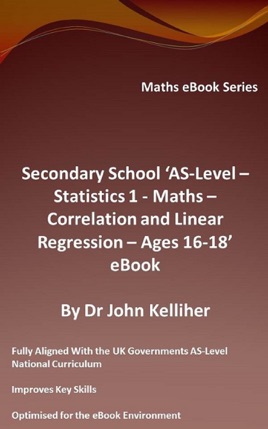• 2,99 €

## Descrizione dell’editore

This eBook introduces the subjects of correlation and linear regression, presenting the relationship between two variables, reviewing the use of scatter diagrams in its review going on to examine the use of the product moment correlation coefficient in correlation, as well as the concept of regression and linear regression in particular, following up with an introduction of Spearman’s rank correlation coefficient. Our eBook goes on to test the students understanding of the subject by setting a number of suitable questions for the student to enjoy.